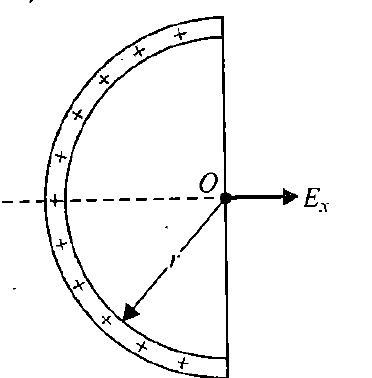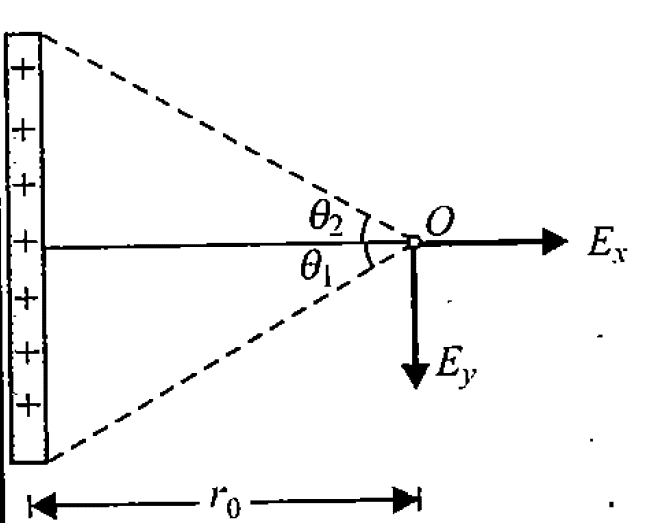# The electric field from its electric potential: semicircle

• iochoa2016
In summary, the electric field is not always positive at the center of a semicircle ring, which is different from what theory predicts.

#### iochoa2016

Homework Statement
Strugling to get the E from its V at the centre of the semicircle.
Relevant Equations
V = k*lambda* pi
pi = 3.1415
lambda = charge density
k = 1 / (4*pi*eo)
According to theory I should be able to get the Electric Field (E) from its pOtential (V) by doing the grad (V) so

E = -grad(V), however, V is contant V = k*lambda* pi which results having E =0, but this is not right. What I am missing??
see figure belowThe answer should be Ex = 2*k*lambda / r

This is how I calculate V:

dV = k * dQ/r = k*r*d(theta)*lambda / r
dV = k*lambda * d(theta)
Integrating from 0 to pi

V = k* lambda * pi.

Last edited:
What makes you say that ##V## is constant ? All you bring forward is an expresssion for (I suppose?) ##V## at the point ##O## ...

##\ ##

•Delta2
k = constant, lambda = constant so V = constant, isn't it?

How did you 'calculate' this ##V## ?

##\ ##

This is how I calculate V:

dV = k * dQ/r = k*r*d(theta)*lambda / r
dV = k*lambda * d(theta)
Integrating from 0 to pi

V = k* lambda * pi.

iochoa2016 said:
k = constant, lambda = constant so V = constant, isn't it?
You are using the expression for the potential at a specific point, but grad is the derivative wrt position. What will be the potential at a nearby point?

•Delta2
found this:
along an axis pointing out the paper passing through the "O",
V = K*lambda*pi * r /(sqrt(r^2+z^2)

iochoa2016 said:
found this:
along an axis pointing out the paper passing through the "O",
V = K*lambda*pi * r /(sqrt(r^2+z^2)
Okay, but you can find ##\vec E## at a point directly. To obtain ##\vec E## from ##-\nabla V##, you need to find ##V## in a neighbourhood of the point. This is very unlikely to be easier.

Yes, I think you are right. Just triying to use energy to work out the Field.

It was easy for the example below, but not so easy for the semicircle ring.iochoa2016 said:
Homework Statement:: Strugling to get the E from its V at the centre of the semicircle.
Relevant Equations:: V = k*lambda* pi
pi = 4.1516
lambda = charge density
k = 1 / (4*pi*eo)
Check your value of ##π## first unless of course you are from Indiana.••BvU and iochoa2016
Did the problem specifically say to use the potential or did it just ask you to derive the field?

The gradient is essentially the partial derivatives, and to be able to calculate a partial derivative correctly you need to know the formula of the function in an interval ##(a,b)## and not just at a specific point (0 in this case) . You need to know the formula for the potential in a subarea of the circle in this case since we have a 2D case and not just at the central point of the circle (0,0).

Last edited: